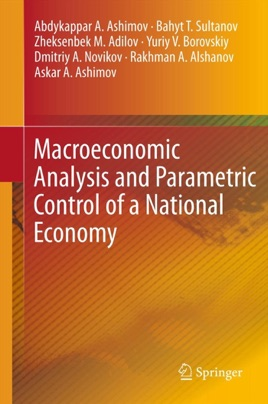• USD 119.99

## Descripción de editorial

​​​​​This is a sequel to the book by Dr. A. Ashimov and his colleagues, Macroeconomic Analysis and Economic Policy Based on Parametric Control. The authors have expanded both the developed mathematical apparatus and the scope of problems and applications stemming from the practice of steering a national economy of a small country in the dynamic environment of the international economic order. The developed theoretical foundation is used in Chapter 1 to suggest a decision support system operating in the framework of state economic policy making. Chapter 2 presents various mathematical models built on the basis of the available statistical data and provides quality assessment of these models. Parametric control problems are being formulated on the basis of these models as problems of mathematical programming, and the obtained solutions are subjected to analyses and interpretations. This is demonstrated by the analysis of the effect of uncontrollable factors on the problem solutions. Chapter 3 is aimed at the modelling and analysis of cyclic phenomena in economics and their structural stability. Chapter 4 presents solutions of specific problems of national economy and analysis and interpretation of their solutions. In summary, the authors formulate comprehensive mathematical models of some critical mechanisms in micro economics previously known only on a qualitative level. They provide vigorous mathematical analysis of the models that justifies their applicability for the formulation of parametric control problems, and the existence of model-based solutions. The complexity of the resultant problems is addressed by the formulation of the appropriate algorithms. The described methodology leads to the development of computer-based decision support systems.​